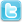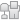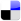ECU hacking -> Kawasaki ZZR1400 / ZX-14 -> petrik k I need your help!!!!!!
Post Info TOPIC: petrik k I need your help!!!!!!

Member

Status: Offline
Posts: 9
Date:
 petrik k I need your help!!!!!! Permalink Petrol k I need your help. Can you rewrite code on the ism lines for a zx12r? Ridge Racer started working on it. Here is the code... A simplified version of the software is... To set trims: IF (Cov3 = Hi) { IF (COV2 = Cyl1) THEN Hi1 = COV1 IF (COV2 = Cyl2) THEN Hi2 = COV1 IF (COV2 = Cyl3) THEN Hi3 = COV1 IF (COV2 = Cyl4) THEN Hi4 = COV1 } IF (Cov3 = Med) { IF (COV2 = Cyl1) THEN Med1 = COV1 IF (COV2 = Cyl2) THEN Med2 = COV1 IF (COV2 = Cyl3) THEN Med3 = COV1 IF (COV2 = Cyl4) THEN Med4 = COV1 } IF (Cov3 = Low) { IF (COV2 = Cyl1) THEN Lo1 = COV1 IF (COV2 = Cyl2) THEN Lo2 = COV1 IF (COV2 = Cyl3) THEN Lo3 = COV1 IF (COV2 = Cyl4) THEN Lo4 = COV1 } To apply trims IF(Throttle = Hi) { IF(Cylinder = 1) THEN Fuel1 = Fuel1 * Hi1 IF(Cylinder = 2) THEN Fuel2 = Fuel2 * Hi2 IF(Cylinder = 3) THEN Fuel3 = Fuel3 * Hi3 IF(Cylinder = 4) THEN Fuel4 = Fuel4 * Hi4 } IF(Throttle = Med) { IF(Cylinder = 1) THEN Fuel1 = Fuel1 * Med1 IF(Cylinder = 2) THEN Fuel2 = Fuel2 * Med2 IF(Cylinder = 3) THEN Fuel3 = Fuel3 * Med3 IF(Cylinder = 4) THEN Fuel4 = Fuel4 * Med4 } IF(Throttle = Lo) { IF(Cylinder = 1) THEN Fuel1 = Fuel1 * Lo1 IF(Cylinder = 2) THEN Fuel2 = Fuel2 * Lo2 IF(Cylinder = 3) THEN Fuel3 = Fuel3 * Lo3 IF(Cylinder = 4) THEN Fuel4 = Fuel4 * Lo4 } What if we rewrote the software to use a single memory location for all settings of COV2 and 3? then their settings become irrelevant IF (Cov3 = Hi) { IF (COV2 = Cyl1) THEN Hi1 = COV1 IF (COV2 = Cyl2) THEN Hi1 = COV1 IF (COV2 = Cyl3) THEN Hi1 = COV1 IF (COV2 = Cyl4) THEN Hi1 = COV1 } IF (Cov3 = Med) { IF (COV2 = Cyl1) THEN Hi1 = COV1 IF (COV2 = Cyl2) THEN Hi1 = COV1 IF (COV2 = Cyl3) THEN Hi1 = COV1 IF (COV2 = Cyl4) THEN Hi1 = COV1 } IF (Cov3 = Lo) { IF (COV2 = Cyl1) THEN Hi1 = COV1 IF (COV2 = Cyl2) THEN Hi1 = COV1 IF (COV2 = Cyl3) THEN Hi1 = COV1 IF (COV2 = Cyl4) THEN Hi1 = COV1 } To apply trims IF(Throttle = Hi) { IF(Cylinder = 1) THEN Fuel1 = Fuel1 * Hi1 IF(Cylinder = 2) THEN Fuel2 = Fuel2 * Hi1 IF(Cylinder = 3) THEN Fuel3 = Fuel3 * Hi1 IF(Cylinder = 4) THEN Fuel4 = Fuel4 * Hi1 } IF(Throttle = Med) { IF(Cylinder = 1) THEN Fuel1 = Fuel1 * Hi1 IF(Cylinder = 2) THEN Fuel2 = Fuel2 * Hi1 IF(Cylinder = 3) THEN Fuel3 = Fuel3 * Hi1 IF(Cylinder = 4) THEN Fuel4 = Fuel4 * Hi1 } IF(Throttle = Low) { IF(Cylinder = 1) THEN Fuel1 = Fuel1 * Hi1 IF(Cylinder = 2) THEN Fuel2 = Fuel2 * Hi1 IF(Cylinder = 3) THEN Fuel3 = Fuel3 * Hi1 IF(Cylinder = 4) THEN Fuel4 = Fuel4 * Hi1 } On 2/18/2012 7:03 AM, Brian Crowell wrote: I was thinking I could permanently ground cos1 to ground with relay or something to put the line in ENABLE mode. Then I figured I could wire a wide band 02 sensor into cov1. 2.5volts no change etc. To solve the cov3 problem I figured I could run a wire from the throttle position sensor to the cov3 plug so the ism line would know what r __________________ Brian Crowell
 Page 1 of 1  sorted by Oldest FirstNewest First